NetworkX

#### Previous topic

spectral_bipartivity

#### Next topic

average_clustering

# clustering¶

clustering(G, nodes=None, mode='dot')[source]

Compute a bipartite clustering coefficient for nodes.

The bipartie clustering coefficient is a measure of local density of connections defined as [R122]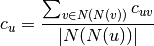where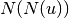are the second order neighbors of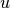in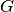excluding, and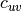is the pairwise clustering coefficient between nodesand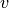.

The mode selects the function for‘dot’: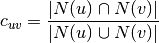‘min’: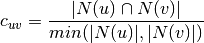‘max’: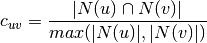Parameters : G : graph A bipartite graph nodes : list or iterable (optional) Compute bipartite clustering for these nodes. The default is all nodes in G. mode : string The pariwise bipartite clustering method to be used in the computation. It must be “dot”, “max”, or “min”. clustering : dictionary A dictionary keyed by node with the clustering coefficient value.

References

 [R122] (1, 2) Latapy, Matthieu, Clémence Magnien, and Nathalie Del Vecchio (2008). Basic notions for the analysis of large two-mode networks. Social Networks 30(1), 31–48.

Examples

>>> from networkx.algorithms import bipartite
>>> G=nx.path_graph(4) # path is bipartite
>>> c=bipartite.clustering(G)
>>> c
0.5
>>> c=bipartite.clustering(G,mode='min')
>>> c
1.0